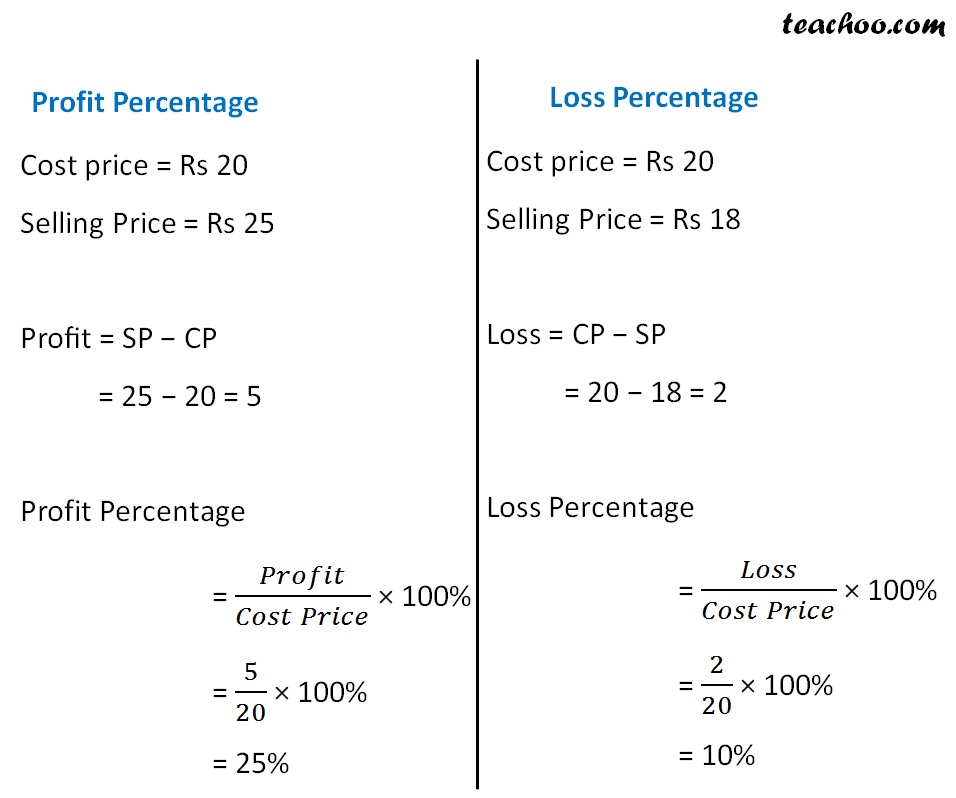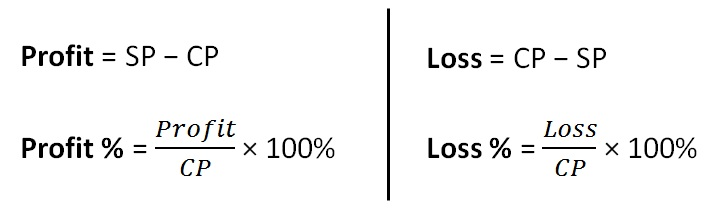Cost price, selling price, profit % , loss%

Chapter 7 Class 7 Comparing Quantities
Concept wise

We can also write Profit or Loss in PercentageSo, we can writeLearn in your speed, with individual attention - Teachoo Maths 1-on-1 Class

### Transcript

Profit & Loss Percentage We can also write Profit or Loss (Link) in Percentage Profit Percentage Cost price = Rs 20 Selling Price = Rs 25 Profit = SP − CP = 25 − 20 = 5 Profit Percentage = 𝑃𝑟𝑜𝑓𝑖𝑡/(𝐶𝑜𝑠𝑡 𝑃𝑟𝑖𝑐𝑒) × 100% = 5/20 × 100% = 25% Loss Percentage Cost price = Rs 20 Selling Price = Rs 18 Loss = CP − SP = 20 − 18 = 2 Loss Percentage = 𝐿𝑜𝑠𝑠/(𝐶𝑜𝑠𝑡 𝑃𝑟𝑖𝑐𝑒) × 100% = 2/20 × 100% = 10% So, we can write Profit = SP − CP Profit % = 𝑃𝑟𝑜𝑓𝑖𝑡/𝐶𝑃 × 100% Loss = CP − SP Loss % = 𝐿𝑜𝑠𝑠/𝐶𝑃 × 100%